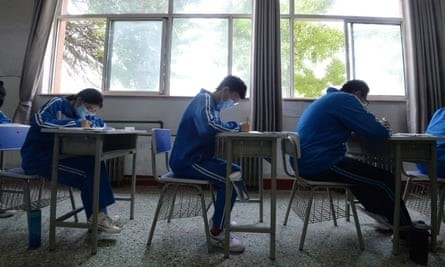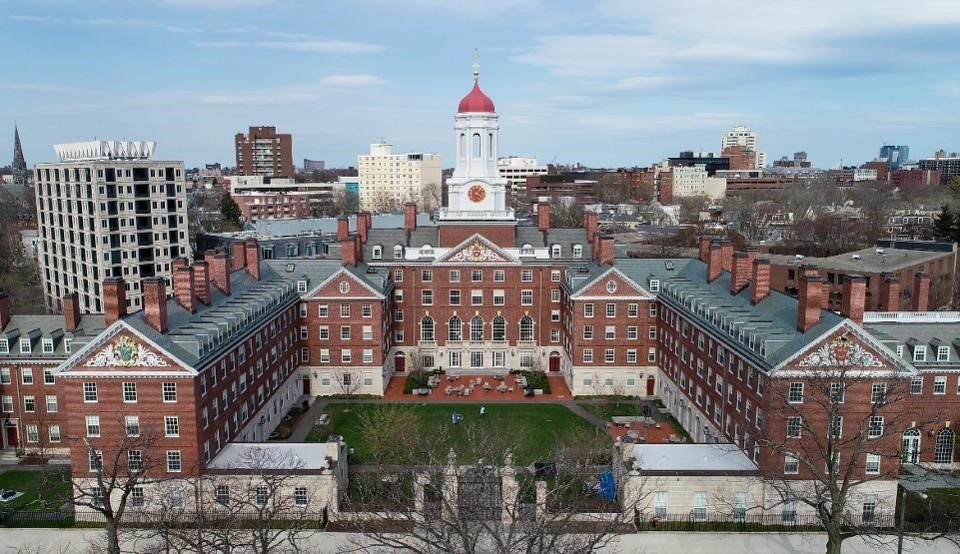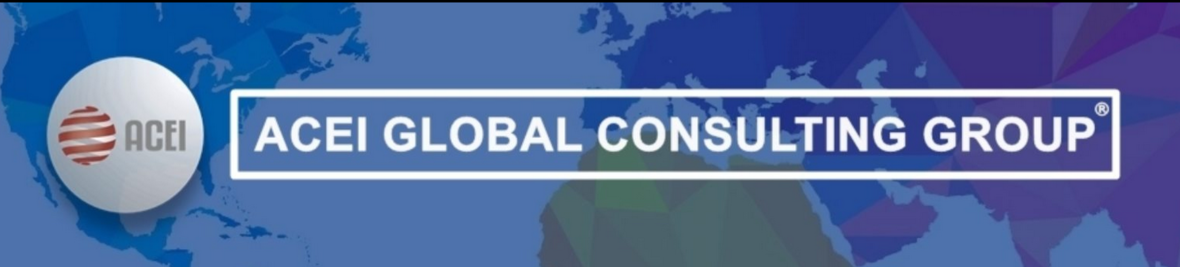# July 2020 - Los Angeles, CA

ACADEMIC CREDENTIALS EVALUATION INSTITUTE, INC. (ACEI)
Founded in 1994, ACEI is a full-service company providing complete and integrated services in-house in the areas of international education research, credential evaluation, and translation. And, through our Global Consulting Group®, we offer our expertise in the following specialties: Media and Branding, Global Pathways, and Diversity, Equity and Inclusion (DEI) to interested institutions and organizations around the globe.

 table div table+table+table div table{width:100%;padding:0}table div table+table+table div table img{width:96.23%;padding:0;float:none}table div table+table+table div table td{width:100%;padding:0 1.88% 18px}/* styles */table div table+table+table+table+table+table div table{width:100%;padding:0}table div table+table+table+table+table+table div table img{width:96.23%;padding:0;float:none}table div table+table+table+table+table+table div table td{width:100%;padding:0 1.88% 18px}/* styles */# ANNOUNCEMENT: ACEI’s TEXAS BRANCH OFFICE

Academic Credentials Evaluation Institute, Inc. (ACEI) is pleased to announce the opening of its branch office in Georgetown, Texas. Our headquarters remain in Los Angeles, CA.

Our branch office in Georgetown, Texas will serve as the processing center for applications and official/original academic documents. Please update your records to include the following address for your applicants to submit their documents:

ACEI, Inc.
723 W UNIVERSITY AVENUE, SUITE 110-151
GEORGETOWN, TX 78626-2663

ACEI’S telephone number 1-310-275-3530, website www.acei-global.org, and email acei@acei-global.org remain unchanged and our client relations team is available to answer any question you and your applicants may have.

Thank you for your continuous support. We look forward to serving you and your domestic and international applicants.

 table div table+table+table+table+table+table+table+table div table{width:100%;padding:0}table div table+table+table+table+table+table+table+table div table img{width:96.23%;padding:0;float:none}table div table+table+table+table+table+table+table+table div table td{width:100%;padding:0 1.88% 18px}/* styles */Image source: Shutterstock

# Granting Temporary Stay to International Graduates

The Australian government is expected to modify requirements for the 485 visa that grants international graduates temporary stay in the country after graduating. To read more, click here.

 table div table+table+table+table+table+table+table+table+table+table+table div table{width:100%;padding:0}table div table+table+table+table+table+table+table+table+table+table+table div table img{width:96.23%;padding:0;float:none}table div table+table+table+table+table+table+table+table+table+table+table div table td{width:100%;padding:0 1.88% 18px}/* styles */Image credit: Photograph: REX/Shutterstock

# Criminalizing College Exam Fraud after Identity Fraud

Chinese lawmakers are seeking to criminalize identity theft in college entrance exams, after revelations that hundreds of students in a single province had their scores stolen or used by others. To read more, click here.

 table div table+table+table+table+table+table+table+table+table+table+table+table+table+table div table{width:100%;padding:0}table div table+table+table+table+table+table+table+table+table+table+table+table+table+table div table img{width:96.23%;padding:0;float:none}table div table+table+table+table+table+table+table+table+table+table+table+table+table+table div table td{width:100%;padding:0 1.88% 18px}/* styles */Image credit: Cairo University (Reuters)

# Universities Adopt the Hybrid Education model

Egypt’s 2020-21 university academic year will begin on Saturday 17 October, with the implementation of a ‘hybrid education’ model aimed at reducing the density of students inside education halls. To read more, click here.

 table div table+table+table+table+table+table+table+table+table+table+table+table+table+table+table+table+table div table{width:100%;padding:0}table div table+table+table+table+table+table+table+table+table+table+table+table+table+table+table+table+table div table img{width:96.23%;padding:0;float:none}table div table+table+table+table+table+table+table+table+table+table+table+table+table+table+table+table+table div table td{width:100%;padding:0 1.88% 18px}/* styles */# 3-Child Policy Pathway to Maitrise

France offers a pathway, via a lottery, to the Maitrise program in Elementary Education to a parent of three children. To read more, click here.

 table div table+table+table+table+table+table+table+table+table+table+table+table+table+table+table+table+table+table+table+table div table{width:100%;padding:0}table div table+table+table+table+table+table+table+table+table+table+table+table+table+table+table+table+table+table+table+table div table img{width:96.23%;padding:0;float:none}table div table+table+table+table+table+table+table+table+table+table+table+table+table+table+table+table+table+table+table+table div table td{width:100%;padding:0 1.88% 18px}/* styles */A promotional banner of the national security law for Hong Kong, in Hong Kong, Tuesday, June 30, 2020. Credit: AP Photo/Kin Cheung

# The New Security Law and its Impact on HK’s Higher Education

Hong Kong’s academic success is quite remarkable for its size and its higher education’s USP has been its openness, internationalization and cosmopolitanism. Could all that be about to change with the imposition of the new security law and what will be the wider impact? To read more, click here.

 table div table+table+table+table+table+table+table+table+table+table+table+table+table+table+table+table+table+table+table+table+table+table+table div table{width:100%;padding:0}table div table+table+table+table+table+table+table+table+table+table+table+table+table+table+table+table+table+table+table+table+table+table+table div table img{width:96.23%;padding:0;float:none}table div table+table+table+table+table+table+table+table+table+table+table+table+table+table+table+table+table+table+table+table+table+table+table div table td{width:100%;padding:0 1.88% 18px}/* styles */Image source: shutterstock

# Committee Looks into Private University Sub-Campuses

Punjab Universities Chancellor and Governor Chaudhry Sarwar has constituted a six-member inquiry committee headed by Quaid-i-Azam University Vice-Chancellor Professor Muhammad Ali to review the quality of education and provision of facilities in the sub-campuses of private universities across the province of Punjab in Pakistan. To read more, click here.

 table div table+table+table+table+table+table+table+table+table+table+table+table+table+table+table+table+table+table+table+table+table+table+table+table+table div table{width:100%;padding:0}table div table+table+table+table+table+table+table+table+table+table+table+table+table+table+table+table+table+table+table+table+table+table+table+table+table div table img{width:96.23%;padding:0;float:none}table div table+table+table+table+table+table+table+table+table+table+table+table+table+table+table+table+table+table+table+table+table+table+table+table+table div table td{width:100%;padding:0 1.88% 18px}/* styles */Image source: Image Hub

# Stringent Conditions for Private Higher Education Institutions

A series of tough requirements will soon be imposed on Rwandan private higher learning institutions and investors in the sector in order to ensure that quality education is not compromised. To read more, click here.

 table div table+table+table+table+table+table+table+table+table+table+table+table+table+table+table+table+table+table+table+table+table+table+table+table+table+table+table div table{width:100%;padding:0}table div table+table+table+table+table+table+table+table+table+table+table+table+table+table+table+table+table+table+table+table+table+table+table+table+table+table+table div table img{width:96.23%;padding:0;float:none}table div table+table+table+table+table+table+table+table+table+table+table+table+table+table+table+table+table+table+table+table+table+table+table+table+table+table+table div table td{width:100%;padding:0 1.88% 18px}/* styles */Image source: Wikipedia

# Universities to Crackdown on Grade Inflation

Universities Minister Michelle Donelan warned higher education institutions across Britain to stop rounding up borderline marks and avoid discounting core or final-year. It means that first-class degrees will be harder to get, after universities agreed on measures to tackle grade inflation. To read more, click here

 table div table+table+table+table+table+table+table+table+table+table+table+table+table+table+table+table+table+table+table+table+table+table+table+table+table+table+table+table+table div table{width:100%;padding:0}table div table+table+table+table+table+table+table+table+table+table+table+table+table+table+table+table+table+table+table+table+table+table+table+table+table+table+table+table+table div table img{width:96.23%;padding:0;float:none}table div table+table+table+table+table+table+table+table+table+table+table+table+table+table+table+table+table+table+table+table+table+table+table+table+table+table+table+table+table div table td{width:100%;padding:0 1.88% 18px}/* styles */Image Credit: Harvard University's Dunster House in Cambridge, MA, on April 11, 2020. (Photo by Blake Nissen for The Boston Globe via Getty Images) BOSTON GLOBE VIA GETTY IMAGES

# No New International Students at Harvard

In a stunning announcement, a Dean of Harvard told first-year international students they could not come to Harvard this fall because the Trump administration has not changed immigration rules on online instruction. To read more, click here

 table div table+table+table+table+table+table+table+table+table+table+table+table+table+table+table+table+table+table+table+table+table+table+table+table+table+table+table+table+table+table+table+table div table{width:100%;padding:0}table div table+table+table+table+table+table+table+table+table+table+table+table+table+table+table+table+table+table+table+table+table+table+table+table+table+table+table+table+table+table+table+table div table img{width:96.23%;padding:0;float:none}table div table+table+table+table+table+table+table+table+table+table+table+table+table+table+table+table+table+table+table+table+table+table+table+table+table+table+table+table+table+table+table+table div table td{width:100%;padding:0 1.88% 18px}/* styles */# Benefits of Studying at a US Community College

Students who take a few courses at community colleges increase their chances of earning a bachelor's degree, according to a new study from the Community College Research Center at Columbia University's Teachers College. To read more, click here

# Senate Republicans’ Proposal for Liability Protection

A proposal by Senate Republicans, being reviewed by the Trump administration, would make it harder for those who are infected with the coronavirus to sue universities, businesses and others. To read more, click here

# North Carolina Law Protects Colleges From COVID-related Lawsuits

A North Carolina law protects colleges from lawsuits related to campus closures as a result of COVID-19. To read more, click here

# New DACA Applications Rejected by Trump Administration

The Trump administration is refusing to process new applications for the Deferred Action for Childhood Arrivals program despite a Supreme Court ruling that required reinstatement of the program. To read more, click here

 table div table+table+table+table+table+table+table+table+table+table+table+table+table+table+table+table+table+table+table+table+table+table+table+table+table+table+table+table+table+table+table+table+table+table div table{width:100%;padding:0}table div table+table+table+table+table+table+table+table+table+table+table+table+table+table+table+table+table+table+table+table+table+table+table+table+table+table+table+table+table+table+table+table+table+table div table img{width:96.23%;padding:0;float:none}table div table+table+table+table+table+table+table+table+table+table+table+table+table+table+table+table+table+table+table+table+table+table+table+table+table+table+table+table+table+table+table+table+table+table div table td{width:100%;padding:0 1.88% 18px}/* styles */Center for Disease Control

World Health Organization

Worldometers

 table div table+table+table+table+table+table+table+table+table+table+table+table+table+table+table+table+table+table+table+table+table+table+table+table+table+table+table+table+table+table+table+table+table+table+table+table div table{width:100%;padding:0}table div table+table+table+table+table+table+table+table+table+table+table+table+table+table+table+table+table+table+table+table+table+table+table+table+table+table+table+table+table+table+table+table+table+table+table+table div table img{width:96.23%;padding:0;float:none}table div table+table+table+table+table+table+table+table+table+table+table+table+table+table+table+table+table+table+table+table+table+table+table+table+table+table+table+table+table+table+table+table+table+table+table+table div table td{width:100%;padding:0 1.88% 18px}/* styles */table div table+table+table+table+table+table+table+table+table+table+table+table+table+table+table+table+table+table+table+table+table+table+table+table+table+table+table+table+table+table+table+table+table+table+table+table+table+table+table+table div table{width:100%;padding:0}table div table+table+table+table+table+table+table+table+table+table+table+table+table+table+table+table+table+table+table+table+table+table+table+table+table+table+table+table+table+table+table+table+table+table+table+table+table+table+table+table div table img{width:96.23%;padding:0;float:none}table div table+table+table+table+table+table+table+table+table+table+table+table+table+table+table+table+table+table+table+table+table+table+table+table+table+table+table+table+table+table+table+table+table+table+table+table+table+table+table+table div table td{width:100%;padding:0 1.88% 18px}/* styles */